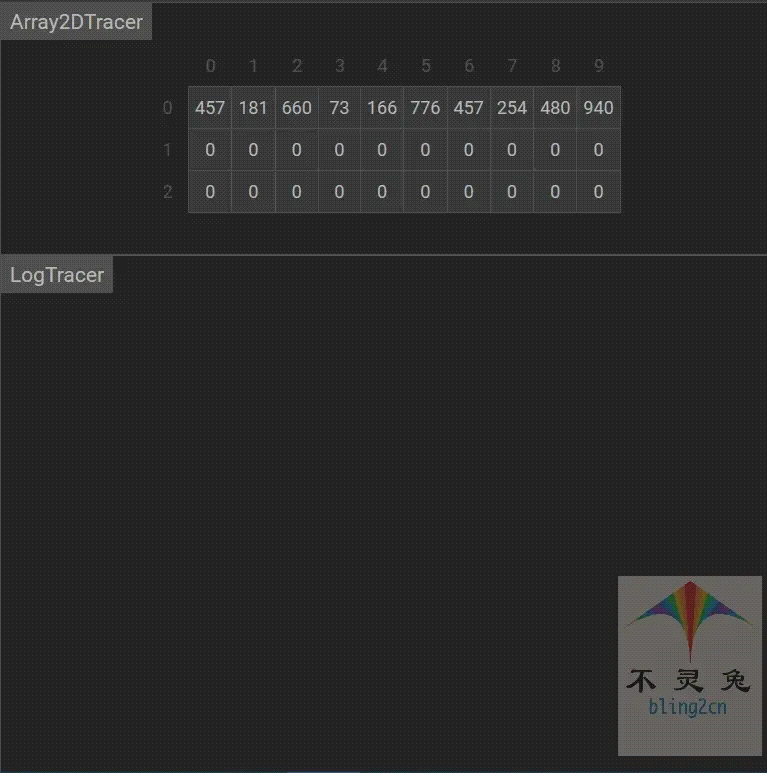# Python implementation · Radix Sort of the top ten sorting algorithms

Posted May 27, 20203 min read

## Introduction

Radix Sort(Radix Sort) is a non-comparative integer sorting algorithm that is an extension of bucket sorting. The basic idea is to unify all the values to be compared into the same digit length, with zeros in front of the shorter digits. Sort in low order first, put them into 10 queues respectively, and then use the first-in first-out principle to collect; then sort in high order, and then collect; and so on, until the highest order, and finally get the sorted sequence. For a set of sequences with small values, the speed is very fast, the time complexity is linear, and the idea is also very clever.

## Algorithm implementation steps

1. Get the maximum number in the array and get the number of digits;
2. Lead zeros in front of the shorter digits;
3. Allocate, start with a single bit, and put it into buckets 0 ~ 9 according to the bit value(0-9);
4. Collect, and then put the data placed in buckets 0 ~ 9 into the array in order;
5. Repeat the 3 ~ 4 process until the highest bit, then the sorting can be completed.

## Python code implementation

``````# radix_sort code implementation

from typing import List

n = len(str(max(arr))) # record the maximum number of digits
for k in range(n):#n round sorting
# Generate 10 lists per round
bucket_list = [[]for i in range(10)]# Because each digit is 0 ~ 9, 10 buckets are created
for i in arr:
# According to the kth place into the bucket
bucket_list [i //(10 ** k)%10].append(i)
# Rearrange the list in the order of the current bucket
arr = [j for i in bucket_list for j in i]
return arr

# Test Data

if __name__ == '__main__':
import random
random.seed(54)
arr = [random.randint(0,100) for _ in range(10)]
print("raw data:", arr)
print("The result of counting and sorting is:", arr_new)

# Output result

Original data:[17, 56, 71, 38, 61, 62, 48, 28, 57, 42]
Counting and sorting results are:[17, 28, 38, 42, 48, 56, 57, 61, 62, 71]``````

## Animated demo## Analysis of Algorithms

• time complexity

Suppose the array to be sorted is \$R \ left \ [1,2, \ cdots, n \ right ]\$, the largest number in the array is \$k \$digits, the base is \$r \$(the decimal base is 10 , Numbers 0 ~ 9, up to 10 buckets are required to map array elements). To process a single digit, you need to map the array elements into \$r \$buckets. After the mapping is completed, you need to collect it. This is equivalent to traversing the entire array. The time complexity of traversing a single digit is \$O(n + r) \$. Therefore, the total time complexity is \$O((n + r) × k) \$.

• Space complexity

During the cardinality sorting process, \$r \$queues need to be opened. In the worst case, a two-dimensional array of \$r × n \$may be used as a bucket, so the space complexity is \$O(r × n) \$.

• Stability

The cardinality sorting process is sorting and collecting separately, and does not affect the relative position of equal elements, so it is stable.

• Overview

Time complexity(average) Time complexity(best) Time complexity(worst) Space complexity Sort by Stability
\$O((n + r) × k) \$ \$O((n + r) × k) \$ \$O((n + r) × k) \$ \$O(r × n) \$ out -place stable